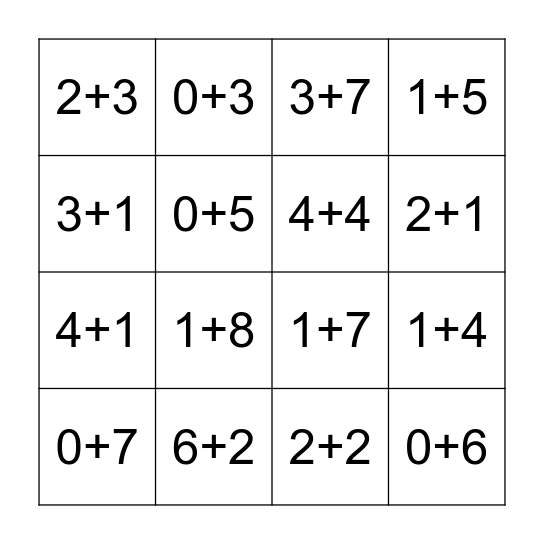This bingo card has 66 words: 0+0, 0+1, 1+0, 0+2, 1+1, 2+0, 0+3, 1+2, 2+1, 3+0, 0+4, 1+3, 2+2, 3+1, 4+0, 0+5, 1+4, 2+3, 3+2, 4+1, 5+0, 0+6, 1+5, 2+4, 3+3, 4+2, 5+1, 6+0, 0+7, 1+6, 2+5, 3+4, 4+3, 5+2, 6+1, 7+0, 0+8, 1+7, 2+6, 3+5, 4+4, 5+3, 6+2, 7+1, 8+0, 0+9, 1+8, 2+7, 3+6, 4+5, 5+4, 6+3, 7+2, 8+1, 9+0, 0+10, 1+9, 2+8, 3+7, 4+6, 5+5, 6+4, 7+3, 8+2, 9+1 and 10+0.

## Play Online